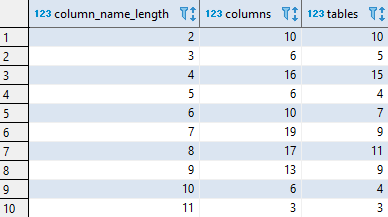## Get column name length distribution in Redshift

Article for:

Query below returns distribution of column name lengths (number of characters).

## Query

``````select length(c.column_name) as column_name_length,
count(*) as columns,
count(distinct c.table_schema || '.' || c.table_name) as tables
from information_schema.columns c
join information_schema.tables t
on t.table_schema = c.table_schema
and t.table_name = c.table_name
where t.table_schema not in ('information_schema', 'pg_catalog')
and t.table_type = 'BASE TABLE'
group by length(c.column_name)
order by length(c.column_name);
``````

## Columns

• column_name_length - lenght in characters of column name
• columns - number of columns with this length
• tables - number of tables that have columns with this name length

## Rows

• One row represents one name lenght (number of characters)
• Scope of rows: each column length that exists in a database
• Ordered by length ascending (from 1 to max)

## Sample results